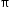# Sample Test Item Preview

This document was generated on CPALMS - www.cpalms.org

### General Information

Related Standards: MAFS.6.RP.1.3
Reporting Category: Ratios & Proportional Relationships
Type: MC: Multiple Choice
Difficulty: N/A
Question:

In a circle, which ratio is equivalent to?

A. radius to area

B. diameter to radius

C. area to circumference

D. circumference to diameter

#### Aligned Standards

Code Description
MAFS.6.RP.1.3: Use ratio and rate reasoning to solve real-world and mathematical problems, e.g., by reasoning about tables of equivalent ratios, tape diagrams, double number line diagrams, or equations.

1. Make tables of equivalent ratios relating quantities with whole-number measurements, find missing values in the tables, and plot the pairs of values on the coordinate plane. Use tables to compare ratios.
2. Solve unit rate problems including those involving unit pricing and constant speed. For example, if it took 7 hours to mow 4 lawns, then at that rate, how many lawns could be mowed in 35 hours? At what rate were lawns being mowed?
3. Find a percent of a quantity as a rate per 100 (e.g., 30% of a quantity means 30/100 times the quantity); solve problems involving finding the whole, given a part and the percent.
4. Use ratio reasoning to convert measurement units; manipulate and transform units appropriately when multiplying or dividing quantities.
5. Understand the concept of Pi as the ratio of the circumference of a circle to its diameter.
(1See Table 2 Common Multiplication and Division Situations)
 Clarifications:Examples of Opportunities for In-Depth Focus When students work toward meeting this standard, they use a range of reasoning and representations to analyze proportional relationships.

Printed On:10/7/2022 10:12:34 PM
Print Page | Close this window